# GitHub in 2013: Fork Events

In this IPython notebook I give an overview of GitHub fork events in 2013 based on data obtained from the GitHub Archive. This is part of a series of posts about GitHub in 2013. The source code of this notebook is available in this GitHub repository, the CSV file with the fork events is not included due to its size. If there is demand, I look into uploading it to a data sharing service, proposals of what service to use are welcome.

## Preliminaries

First load the necessary packages, set a global footer text and a limit for the charts below.

In :
```import datetime
import itertools

import pandas as pd
import numpy as np
import matplotlib.pyplot as plt

from utils import graphs

limit = 20
footer = 'Data: githubarchive.org - Source: coderstats.github.io'
```

Read the fork events from a compressed CSV file, set the first column as the index and specify, that it is a date. Also add a Count column and set its values to 1 for aggregating events.

In :
```df_forks = pd.read_csv('csv/githubarchive/2013/fork_events.csv.bz2', compression='bz2', index_col=0, parse_dates=, low_memory=False)
df_forks['Count'] = 1
```
Out:
Actor Actor Type Repo Forks Repo Language Repo Name Repo Owner Repo is Fork Count
2013-01-01 08:00:43 msnrkjwr User 115 Java infinispan infinispan False 1
2013-01-01 08:01:08 SnowCat6 User 28 NaN android_prebuilt_toolchains DooMLoRD False 1
2013-01-01 08:01:06 SrikanthKrish User 254 Shell OpenELEC.tv OpenELEC False 1
2013-01-01 08:02:25 songlipeng2003 User 100 JavaScript nanoScrollerJS jamesflorentino False 1
2013-01-01 08:02:32 trongtran User 35 Python sublime-jsdocs spadgos False 1

5 rows × 8 columns

Forks can be created by users and organizations, below we see how the user type distribution looks like.

In :
```df_forks['Actor Type'].value_counts().plot(kind='bar', rot=0, title='Forks by user type')
plt.show()
```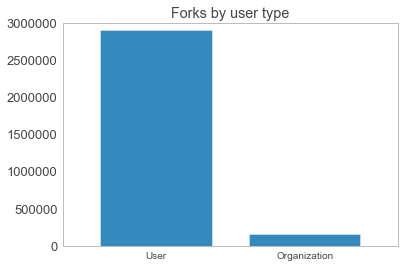## Repositories by forks

Let's find out which repositories were forked most often in 2013. Since repo names are not unique across users, I add a column `Repo Path` composed of user and repo names to have a unique identifier and a column to use for labels.

In :
```df_forks['Repo Path'] = df_forks['Repo Owner'] + '/' + df_forks['Repo Name']
```

Now aggregate the repos grouping by the `Repo Path` column and summing up the `Count` values and plot the most forked repos in a horizontal bar chart.

In :
```repos_grouped = df_forks.groupby('Repo Path')['Count'].sum()
repos_grouped.sort()
top_repos = repos_grouped.tail(limit)

graphs.barh(top_repos.index,
top_repos,
'img/%d-most-forked-github-repos-2013.png' % limit,
figsize=(12, limit / 2),
title='%d most forked GitHub repos in 2013' % limit,
footer=footer)
```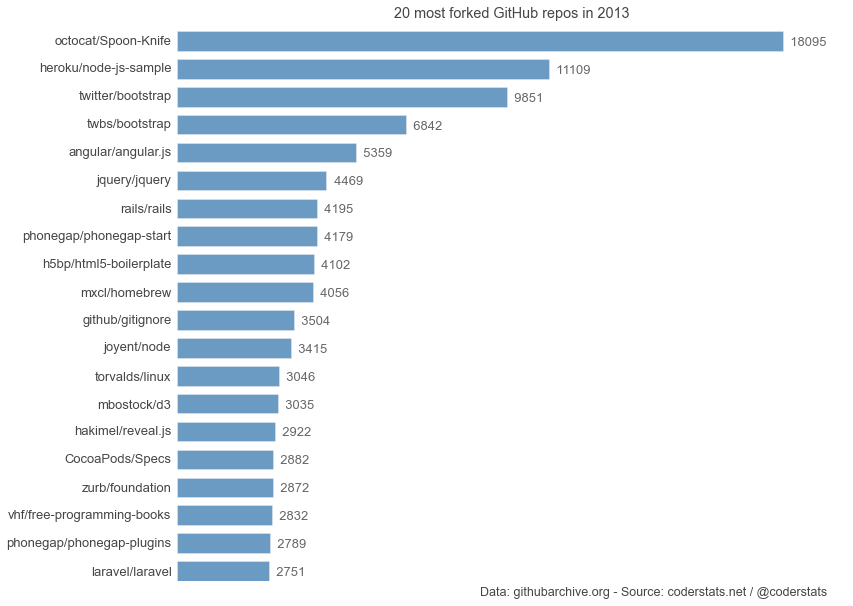Spoon-Knife is GitHub's example repo on the Fork a Repo help page. Apart from that it, is not all that interesting, except maybe for the hidden easter egg (think Konami).

The Heroku node-js-sample is a barebones Node.js app using the Express framework. With so many buzz words in one short sentence it had to be a success, at least for a certain time frame, as we'll see further below.

Place 3 and 4 are actually the same project, bootstrap was moved from Twitter to a dedicated organization twbs, which makes sense, since the project founders Mark Otto and Jacob Thornton left their jobs at Twitter.

Given the noncompetitive nature of the Spoon-Knife project and adding up twitter/bootstrap and twbs/bootstrap, bootstrap was the most forked "real" software project in 2013 by far.

## Distribution of fork counts by repositories

To get a better sense of how "rare" these popular projects are on GitHub in relation to the total number of projects, look at the Histograms below. The 1st one shows the whole distribution using a linear scale, the 2nd the whole distributions on a log scale, and the 3rd repos with up to a 1000 forks in 2013 on a log scale.

In :
```fig, axes = plt.subplots(nrows=1, ncols=3, figsize=(15, 5))

repos_grouped.hist(bins=100, ax=axes)
repos_grouped.hist(bins=100, log=True, ax=axes)
repos_grouped[repos_grouped < 1000].hist(bins=100, log=True, ax=axes)
plt.show()
```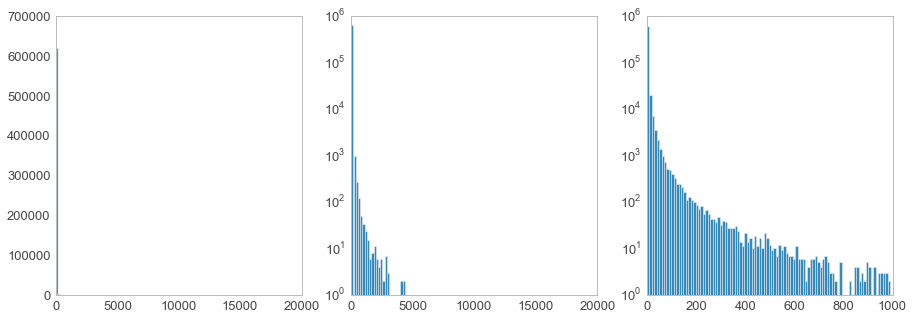## Most forked repositories over time

Next let's look at how the amount of forks of the most forked repositories has evolved over time. To do so plot a timeline for each of the top repos with fork counts grouped by date.

In :
```top = top_repos[::-1]

fig, axes = plt.subplots(nrows=limit / 2, ncols=2, sharex=True)
fig.suptitle('GitHub top repos by forks over time in 2013', y=1.01, fontsize=14)
fig.set_figheight(limit)
fig.set_figwidth(14)

for idx, coords in enumerate(itertools.product(range(limit / 2), range(2))):
ax = axes[coords, coords]
repo = top.index[idx]
repo_forks = df_forks[df_forks['Repo Path'] == repo]
repo_forks['date'] = repo_forks.index.date
grouped = repo_forks.groupby('date').sum()
grouped['Count'].plot(ax=ax, legend=False, rot=45)
ax.set_title(repo)
ax.set_xlabel('', visible=False)

fig.text(0, 0, footer, fontsize=12)
fig.tight_layout()
plt.show()
```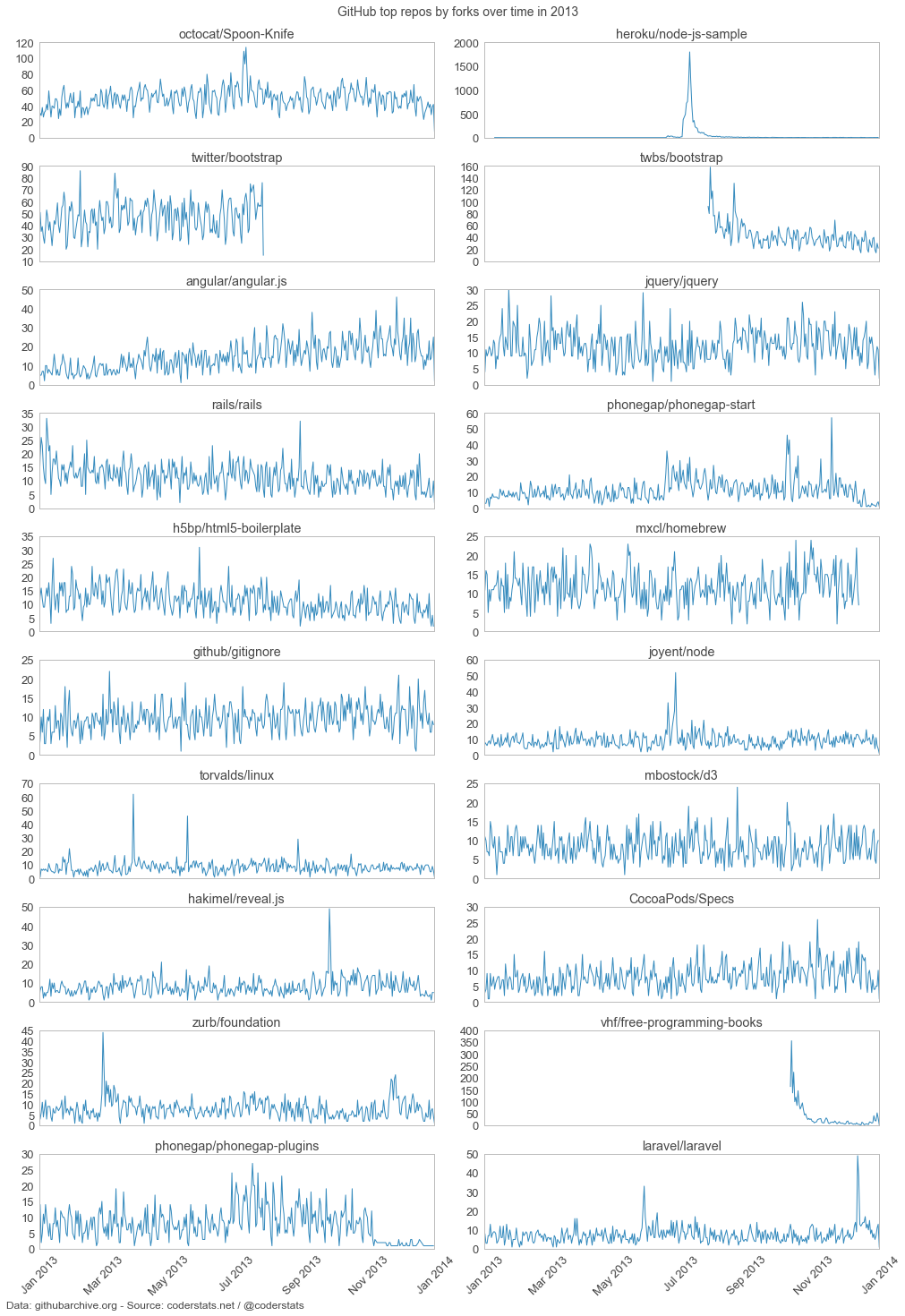As with other timelines we saw in previous github archive posts there is quite some variation between work days and weekends. Moreover, we see spikes, which are most likely to be caused by increased exposure of the project outside of GitHub, e. g. Hacker News, Reddit et al.

The most extreme spike occurs in the heroku/node-js-sample graph. Looking at the project's commit history most of the development occurred in July 2013 around the time of that huge increase in popularity. I assume that there was some kind of announcement by Heroku, which was spread though social networks and news sites, but I haven't found anything concrete.

## Languages by forks

It is no secret that JavaScript is the most popular language on GitHub based on the number of existing repositories. Let's see if this is also true for forks of repositories. A few things to bear in mind though are:

• Many repositories contain code written in multiple languages, the one that has the highest share is represented as the main language, so if JavaScript has 49% and Python 48% and Shell 3%, JavaScript "wins".
• GitHub's language detection does have flaws
• I assume, based on experience not on numbers, that more projects include frontend libraries like jQuery in their repos than backend libraries, which would benefit JS regarding byte count.
In :
```langs_grouped = df_forks.groupby('Repo Language')['Count'].sum()
langs_grouped.sort()
top_langs = langs_grouped.tail(limit)

graphs.barh(top_langs.index,
top_langs,
'img/%d-top-languages-forks-github-2013.png' % limit,
figsize=(12, limit / 2),
title='%d top languages by forks on GitHub in 2013' % limit,
footer=footer)
```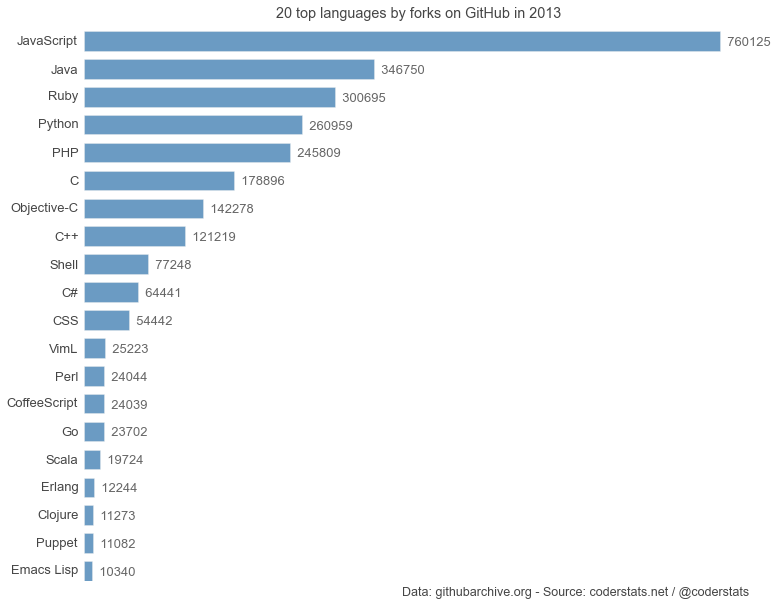Unsurprisingly, JavaScript ranks no. 1, I did not expect such a huge gap between runner-up Java though. Also I did not expect Java to come in 2nd.

## Top languages by forks timelines

As for the repos, let's look at forks by languages over time.

In :
```top = top_langs[::-1]

fig, axes = plt.subplots(nrows=limit / 2, ncols=2, sharex=True)
fig.suptitle('GitHub top languages by forks over time in 2013', y=1.01, fontsize=14)
fig.set_figheight(limit)
fig.set_figwidth(14)

for idx, coords in enumerate(itertools.product(range(limit / 2), range(2))):
ax = axes[coords, coords]
lang = top.index[idx]
lang_forks = df_forks[df_forks['Repo Language'] == lang]
lang_forks['date'] = lang_forks.index.date
grouped = lang_forks.groupby('date').sum()
grouped['Count'].plot(ax=ax, legend=False, rot=45)
ax.set_title(lang)
ax.set_xlabel('', visible=False)

fig.text(0, 0, footer, fontsize=12)
fig.tight_layout()
plt.show()
```